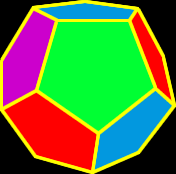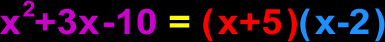# Online Math Dictionary: F

Easy to understand math definitions for K-Algebra mathematics
Just scroll down or click on the word you want and I'll scroll down for you!

 face of a polyhedron factor in arithmetic factor in algebra factorial Fibonacci numbers finite formula fractal fraction function

 Face of a PolyhedronA face of a polyhedron is one of the sides.  Example:  In the dodecahedron on the right, we can see one green face, one purple face, two red facesand two blue faces.  (There are six more faces on the back side that we can't see.)  For  more info on polyhedra, check out myPolyhedra Gallery.Factor in Arithmetic
A factor in arithmetic is one of the two or three (or more) things we multiply together to get our answer.  In the examples below, the factors are the blueand red numbers.
Examples:    6  2 x 3        120 = 4 x 5 x 6